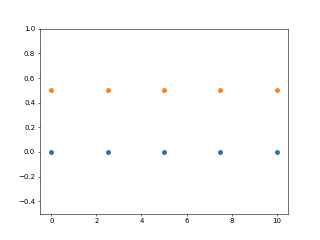# numpy之linspace()函数使用详解

## linspace() 函数

Numpy通常可以使用`numpy.arange()`生成序列，但是当我们使用浮点参数时，可能会导致精度损失，这可能会导致不可预测的输出。为了避免由于浮点精度而造成的任何精度损失，numpy在`numpy.linspace()`为我们提供了一个单独的序列生成器，如果您已经知道所需的元素数，则这是首选。 但是通常使用带有适当参数的`linspace()``arange()`可以得到相同的输出，因此可以为同一任务选择两者。

import numpy as np

import matplotlib.pyplot as plt

y = np.zeros(5)

x1 = np.linspace(0, 10, 5)

x2 = np.linspace(0, 10, 5)

plt.plot(x1, y, 'o')

plt.plot(x2, y + 0.5, 'o')

plt.ylim([-0.5, 1])

plt.show()• start -> Starting point (included) of the rangestart ->范围的起点（包括）
• end -> Endpoint (included) of the rangeend ->范围的端点（包括）
• num -> Total number of points in the sequencenum >序列中的总点数

import numpy as np

a = np.linspace(0.02, 2, 10)

print('Linear Sequence from 0.02 to 2:', a)

print('Length:', len(a))

Linear Sequence from 0.02 to 2: [0.02 0.24 0.46 0.68 0.9  1.12 1.34 1.56 1.78 2.  ]

Length: 10

## endpoint 关键字参数

import numpy as np

a = np.linspace(0.02, 2, 10, endpoint=False)

print('Linear Sequence from 0.02 to 2:', a)

print('Length:', len(a))

Linear Sequence from 0.02 to 2: [0.02  0.218 0.416 0.614 0.812 1.01  1.208 1.406 1.604 1.802]

Length: 10

## retstep 关键字参数

import numpy as np

a = np.linspace(0.02, 2, 10, retstep=True)

print('Linear Sequence from 0.02 to 2:', a)

print('Length:', len(a))

Linear Sequence from 0.02 to 2: (array([0.02, 0.24, 0.46, 0.68, 0.9 , 1.12, 1.34, 1.56, 1.78, 2.  ]), 0.22)

Length: 2

## axis 关键字参数

import numpy as np

p = np.array([[1, 2], [3, 4]])

q = np.array([[5, 6], [7, 8]])

r = np.linspace(p, q, 3, axis=0)

print(r)

s = np.linspace(p, q, 3, axis=1)

print(s)

array([[[1., 2.],

[3., 4.]],

[[3., 4.],

[5., 6.]],

[[5., 6.],

[7., 8.]]])

array([[[1., 2.],

[3., 4.],

[5., 6.]],

[[3., 4.],

[5., 6.],

[7., 8.]]])

0 人点赞